# pylops.FirstDerivative#

class pylops.FirstDerivative(dims, axis=-1, sampling=1.0, kind='centered', edge=False, order=3, dtype='float64', name='F')[source]#

First derivative.

Apply a first derivative using a multiple-point stencil finite-difference approximation along axis.

Parameters
dims

Number of samples for each dimension

axisint, optional

New in version 2.0.0.

Axis along which derivative is applied.

samplingfloat, optional

Sampling step $$\Delta x$$.

kindstr, optional

Derivative kind (forward, centered, or backward).

edgebool, optional

Use reduced order derivative at edges (True) or ignore them (False). This is currently only available

for centered derivative

orderint, optional

New in version 2.0.0.

Derivative order (3 or 5). This is currently only available for centered derivative

dtypestr, optional

Type of elements in input array.

namestr, optional

New in version 2.0.0.

Name of operator (to be used by pylops.utils.describe.describe)

Notes

The FirstDerivative operator applies a first derivative to any chosen direction of a multi-dimensional array using either a second- or third-order centered stencil or first-order forward/backward stencils.

For simplicity, given a one dimensional array, the second-order centered first derivative is:

$y[i] = (0.5x[i+1] - 0.5x[i-1]) / \Delta x$

while the first-order forward stencil is:

$y[i] = (x[i+1] - x[i]) / \Delta x$

and the first-order backward stencil is:

$y[i] = (x[i] - x[i-1]) / \Delta x$

Formulas for the third-order centered stencil can be found at this link.

Attributes
shapetuple

Operator shape

explicitbool

Operator contains a matrix that can be solved explicitly (True) or not (False)

Methods

 __init__(dims[, axis, sampling, kind, edge, ...]) adjoint() apply_columns(cols) Apply subset of columns of operator cond([uselobpcg]) Condition number of linear operator. conj() Complex conjugate operator div(y[, niter, densesolver]) Solve the linear problem $$\mathbf{y}=\mathbf{A}\mathbf{x}$$. dot(x) Matrix-matrix or matrix-vector multiplication. eigs([neigs, symmetric, niter, uselobpcg]) Most significant eigenvalues of linear operator. matmat(X) Matrix-matrix multiplication. matvec(x) Matrix-vector multiplication. reset_count() Reset counters rmatmat(X) Matrix-matrix multiplication. rmatvec(x) Adjoint matrix-vector multiplication. todense([backend]) Return dense matrix. toimag([forw, adj]) Imag operator toreal([forw, adj]) Real operator tosparse() Return sparse matrix. trace([neval, method, backend]) Trace of linear operator. transpose()

## Examples using pylops.FirstDerivative#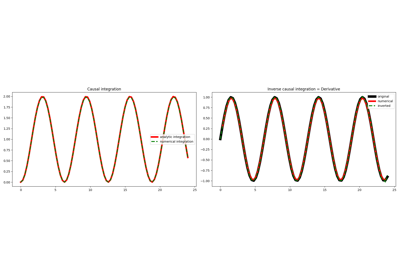Causal Integration

Causal Integration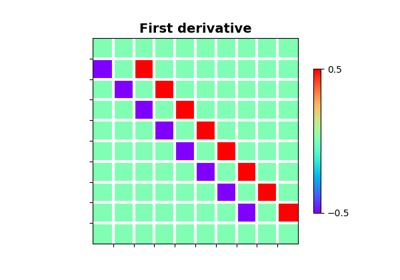Derivatives

Derivatives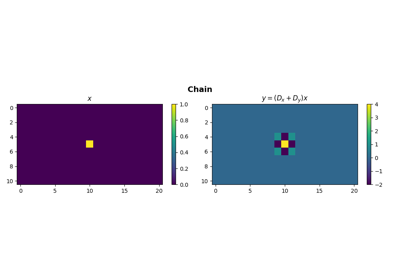Operators concatenation

Operators concatenation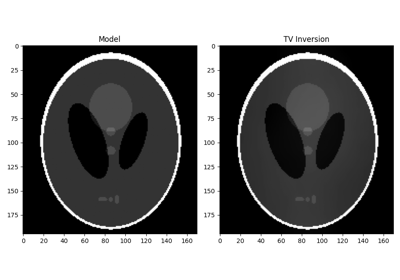Total Variation (TV) Regularization

Total Variation (TV) Regularization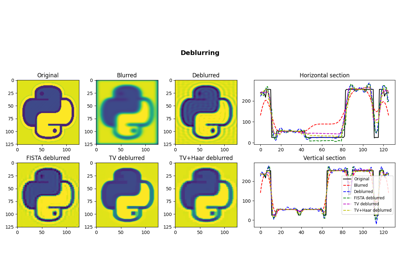05. Image deblurring

05. Image deblurring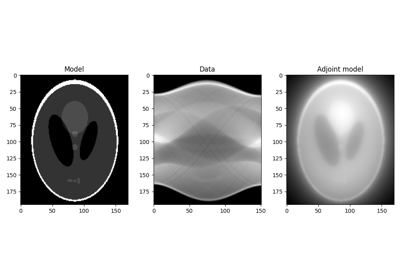16. CT Scan Imaging

16. CT Scan Imaging